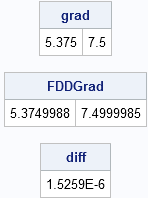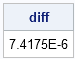I previously wrote about using SAS/IML for nonlinear optimization, and demonstrated optimization by maximizing a likelihood function. Many well-known optimization algorithms require derivative information during the optimization, including the conjugate gradient method (implemented in the NLPCG subroutine) and the Newton-Raphson method (implemented in the NLPNRA method).

You should specify analytic derivatives whenever you can, because they are more accurate and more efficient than numerical derivatives. Computing a derivative is not hard, but it is important to get it right. A mistake in the coding of derivative information can difficult to find, and can lead to wrong answers in the optimization.

That's why I suggest two techniques to make sure that derivatives are correct:

• Use symbolic derivative software to compute complex derivatives.
• Use finite difference computations to check that the derivatives are correct. In SAS/IML software, you can use the NLPFDD subroutine to approximate derivatives with finite-differences.

### Example: Derivatives in MLE estimation

Suppose that you have a real-valued function of several variables. To optimize the function, you might want to compute the gradient function, which is the vector of first derivatives. (The gradient is identically zero at local optima.)

To be concrete, let's reuse the log-likelihood example from my previous blog post. The following SAS/IML module defines the log-likelihood function for fitting a normal density curve to data. Given a data vector (x1, x2, ..., xn), the function can be used to find parameters (μ, σ) such that the data are most likely to be a sample from a normal distribution with those parameters.

```proc iml; /* the log-likelihood function for the normal distribution N(mu, sigma) */ start LogLik(param) global (x); mu = param; sigma2 = param##2; n = nrow(x); c = x - mu; f = -n/2*log(sigma2) - 1/2/sigma2*sum(c##2); return ( f ); finish;```

### Tip 1: Compute symbolic derivatives

The previous log-likelihood function is simple enough that you can manually compute the derivatives of the function with respect to the parameters mu and sigma. However, you might be interested in knowing that there are online tools that you can use to compute symbolic derivatives. The one I use is Wolfram Alpha, which is powered by the same software as Mathematica®. In Mathematica, the D operator is used to compute derivatives. The same operator works in Wolfram Alpha. So, for example, to compute the derivative with respect to sigma, you can type
D[ -n/2*log(sigma^2) - 1/2/sigma^2*Sum[(x_i-mu)^2], sigma]
and Wolfram Alpha will return the derivative
-n/sigma + Sum[(x_i-mu)^2] / sigma^3

I was less successful computing the derivative with respect to mu. Perhaps the Sum[] function confused the software. However, you can take the derivative of the quantity inside the summation:
D[ -1/2/sigma^2*(x_i-mu)^2, mu]
and Wolfram Alpha will return the derivative
(x_i-mu) / sigma^2

The gradient of the log-likelihood function is therefore as follows:

```start GradLogLik(param) global (x); mu = param; sigma = param; sigma2 = sigma##2; n = nrow(x); c = x - mu; dfdmu = sum(c) / sigma2; dfdsigma = -n/sigma + sum(c##2)/sigma##3; return ( dfdmu || dfdsigma ); finish;```

### Tip 2: Use the NLPFDD subroutine to check derivatives

Is the gradient function programmed correctly? Let's find out by calling the GradLogLik function and also the NLPFDD subroutine, which will compute a finite difference approximation to the derivatives of the LogLik function:

```/* Use the NLPFDD subroutine to check analytic derivatives */ use Sashelp.Iris; read all var {SepalWidth} into x; close Sashelp.Iris;   p = {30 4}; /* evaluate derivative at this (mu, sigma) */ grad = GradLogLik(p); call nlpfdd(func, FDDGrad, hessian, "LogLik", p); diff = max(abs(grad - FDDGrad)); print grad, FDDGrad, diff;```Success! The gradient from the GradLogLik function and the finite difference approximation (in the FDDGrad vector) are essentially the same. Of course, we might have gotten lucky; we evaluated the derivative only at a single set of parameter values. If you want to be extra cautious, the following statements use the Define2DGrid module to generate a whole grid of parameter values. The exact gradient and the finite difference derivatives are evaluated at each of these parameter values.

```/* optional: evaluate derivatives on a grid */ params = Define2DGrid( 27, 36, 11, 3, 6, 11 ); exactgrad = j(nrow(params),2); fddgrad = j(nrow(params),2); do i = 1 to nrow(params); exactgrad[i,] = GradLogLik( params[i,] ); call nlpfdd(func, grad, hessian, "LogLik", params[i,] ); fddgrad[i,] = grad; end; diff = max(abs( exactgrad - fddgrad )); print diff;```This was probably more work than was necessary, but it shows that the analytical derivatives and the finite difference derivatives agree to five decimal places. I am now confident that my analytical derivatives are correct.

ShareDistinguished Researcher in Computational Statistics

Rick Wicklin, PhD, is a distinguished researcher in computational statistics at SAS and is a principal developer of SAS/IML software. His areas of expertise include computational statistics, simulation, statistical graphics, and modern methods in statistical data analysis. Rick is author of the books Statistical Programming with SAS/IML Software and Simulating Data with SAS.

1.Hi Rick,

I'm doing a very basic model using SAS/IML for nonlinear optimization by maximizing the likelihood function. Below is my code. It turns out that the model does not converge. The model do converge if I run the it with proc mixed. I was trying to figure out why this happened but I couldn't find out the reason. It is appreciated if you could take a look of my code and let me know what's wrong with it. Thanks in advance!

proc iml;
use x;
use y;
use nsub;
use ntime;
use initial;

nrun=0;
/***loglikelihood funtion***/
start loglik(parameters) global(x,y,nsub,ntime,nrun);
beta=parameters[1:2];
print beta;
rho=parameters;
print rho;
sigma2=parameters;
print sigma2;

ll=0;
ind=1;
do while (ind<=nsub);
xi=x[(ind-1)*ntime+1:ind*ntime,];
yi=y[(ind-1)*ntime+1:ind*ntime];
pi=constant('PI');

/*remove missing values (monotone missing)*/
if (yi[nrow(yi)]=.) then do; /*if yi is not complete*/
j=nrow(yi);
do while (yi[j-1]=.);
j=j-1;
end;
di=j;
yobs=yi[1:di-1];
end;
else do;
yobs=yi;
end;
ll1=0;
ni=nrow(yobs);
mui=xi[1:ni,]*beta;
help1=0:(ni-1);
help2=shape(help1,ni,ni);
h=help2-t(help2);
h=rho##abs(h); /*elementwise power*/
vi=sigma2*h;
ll1=-(ni/2)*log(2*pi)-0.5*log(det(vi))-0.5*t(yobs-mui)*inv(vi)*(yobs-mui);
/*calculate ll*/
ll=ll+ll1;
print ll;
ind=ind+1;
end;
print ll;
nrun=nrun+1;
file log;
put nrun;
return(ll);
finish loglik;

opt={1 11};
con={. . -1 0 ,
. . 1 . };
call nlpnrr(rc, xr, "loglik", initial, opt, con);
inf=-hessian;
covar=inv(inf);
var=vecdiag(covar);
stde=sqrt(var);
create result var {est stde};
append;
quit;

2.I am trying to code a very difficult equation into SAS and see if it can derive derivatives and integrals for me. There are no constants in my equation, just 9 variables. I'm only interested in one of the variables. I'm hoping SAS could also help simplify the equation and solve for the one variable I'm interested in in terms of the other variables. Would you by chance know if this would work? Or what sites/concepts should I look up to figure out how to go about this?
Thanks!!

•Are you able to use the equation to solve for the variable you want in terms of the other variables? SAS/IML has routines for numerical derivatives, but you need to have the equation in the form y = f(x1,x2,...,x8). If you are having problems algebraically inverting an equation, you might try a symbolic algebra package, such as Mathematica. I suggest you post a question to the SAS Support Communities at https://communities.sas.com/ and supply for details about your problem. Choose the "SAS/IML Software and Matrix Computations" board.

•Rick,

Thank you for your response. I believe I can narrow down my complicated equation so I have my variable of interest as the 'y' and my other variables on the other side. There are 8 variables on the other side of y and I believe I'm going to need to take the derivative of all of them separately. I've been reading up on SAS and how to go about doing this, but I'm not sure of the code to use. Would i use the GETDER(Art,Fm) for example? The derivative of Art (which is arterial concentration-my y value), with respect to Fm (derivative of mammary blood flow-which is one of the other 8 variables)?

•No. GETDER is in PROC MODEL, but not in SAS/IML. The optimization methods in SAS/IML will automatically take finite difference derivatives if you don't supply a function that computes derivatives.

The comment field of a blog post is not a good environment to provide assistance for questions. Please post to the Communities.